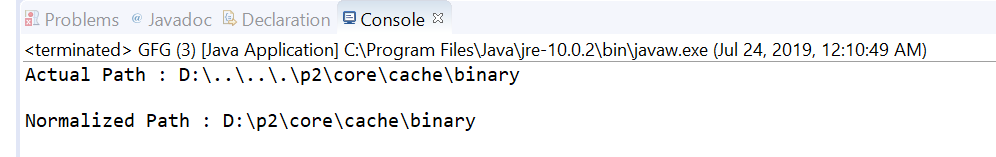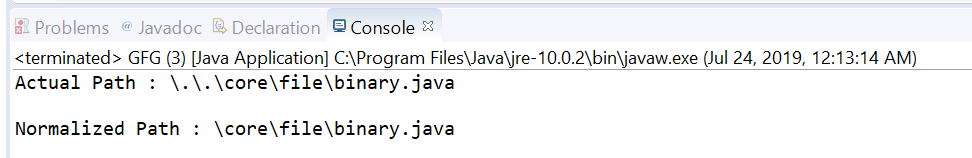# Path normalize() method in Java with Examples

• Last Updated : 30 Jul, 2019

The normalize() method of java.nio.file.Path used to return a path from current path in which all redundant name elements are eliminated.
The precise definition of this method is implementation dependent and it derives a path that does not contain redundant name elements. In many file systems, the “.” and “..” are special names indicating the current directory and parent directory. In those cases all occurrences of “.” are considered redundant and If a “..” is preceded by a non-“..” name then both names are considered redundant.

Syntax:

```Path normalize()
```

Parameters: This method accepts nothing. It is parameter less method.

Return value: This method returns the resulting path or this path if it does not contain redundant name elements; an empty path is returned if this path does not have a root component and all name elements are redundant.

Below programs illustrate normalize() method:
Program 1:

 `// Java program to demonstrate``// java.nio.file.Path.normalize() method`` ` `import` `java.nio.file.*;`` ` `public` `class` `GFG {``    ``public` `static` `void` `main(String[] args)``    ``{`` ` `        ``// create object of Path``        ``// In this example \\.. starts with non".."``        ``// element``        ``Path path``            ``= Paths.get(``"D:\\..\\..\\.\\p2\\core"``                        ``+ ``"\\cache\\binary"``);`` ` `        ``// print actual path``        ``System.out.println(``"Actual Path : "``                           ``+ path);`` ` `        ``// normalize the path``        ``Path normalizedPath = path.normalize();`` ` `        ``// print normalized path``        ``System.out.println(``"\nNormalized Path : "``                           ``+ normalizedPath);``    ``}``}`

Output:Program 2:

 `// Java program to demonstrate``// java.nio.file.Path.normalize() method`` ` `import` `java.nio.file.*;`` ` `public` `class` `GFG {``    ``public` `static` `void` `main(String[] args)``    ``{`` ` `        ``// create object of Path``        ``Path path``            ``= Paths.get(``"\\.\\.\\core"``                        ``+ ``"\\file\\binary.java"``);`` ` `        ``// print actual path``        ``System.out.println(``"Actual Path : "``                           ``+ path);`` ` `        ``// normalize the path``        ``Path normalizedPath = path.normalize();`` ` `        ``// print normalized path``        ``System.out.println(``"\nNormalized Path : "``                           ``+ normalizedPath);``    ``}``}`

Output:My Personal Notes arrow_drop_up